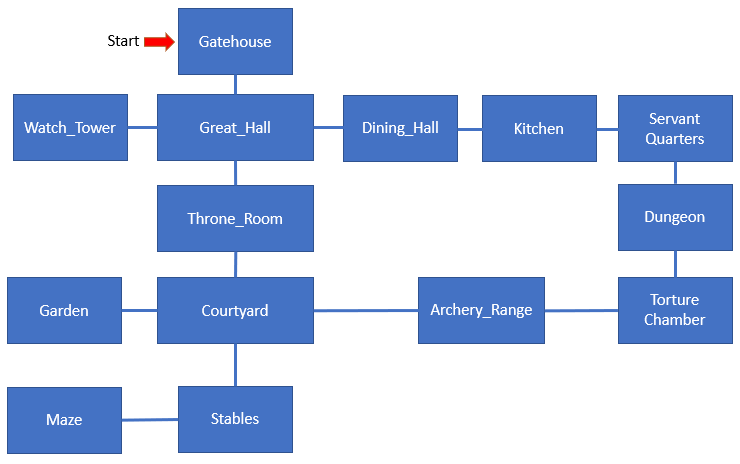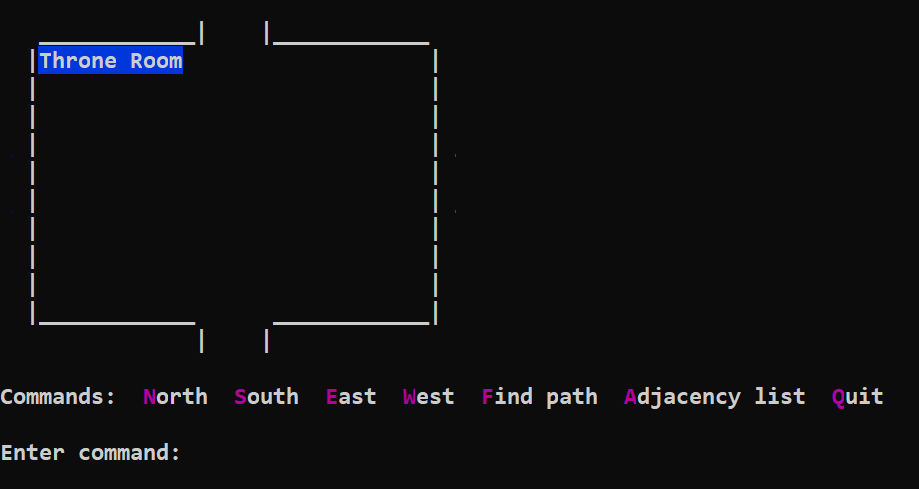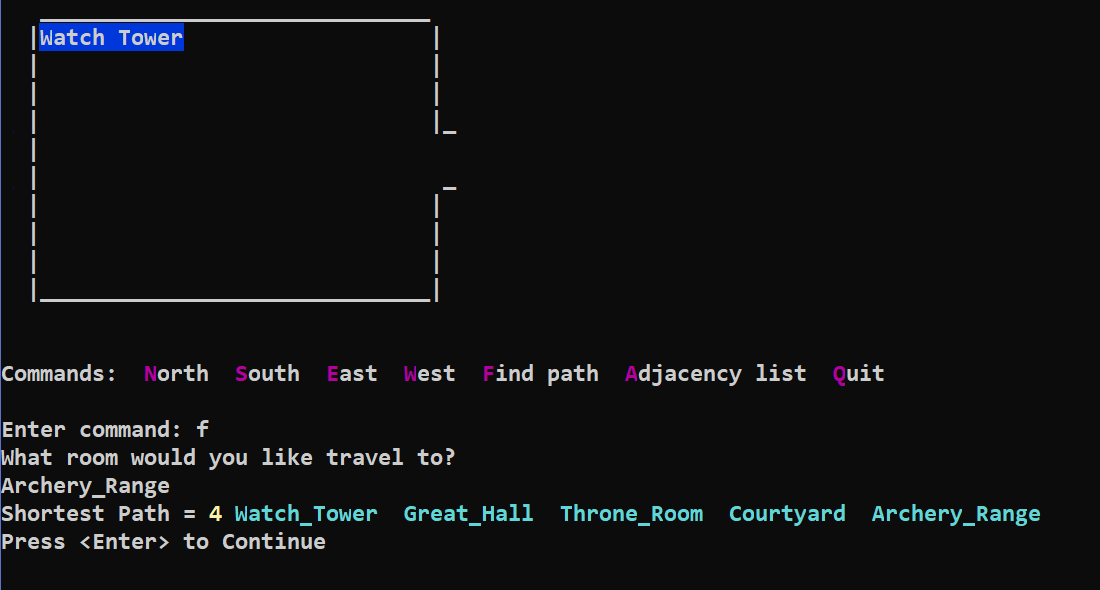Create a complex of connected rooms.  Read in the vertices and edges from files.  Allow the user to move around the complex by going North, South, East, and West.  Implement Dijkstra's algorithm to find the shortest path to a user selected room.  Have the option to show the adjacency list after running Dijkstra's.  Create a drawing of your complex.  Upload the following files to Ecampus:  (1) Drawing of complex, (2) Java source code, (3) vertex.txt, (4) edge.txt

1. Create a drawing of your complex.  It should have multiple paths to some rooms.  You can use PowerPoint for this.2. Create vertex.txt and edge.txt

 vertex.txt contains room names: GateHouse Great_Hall Dining_Hall Throne_Room edge.txt contains room connections: GateHouse South Great_Hall Great_Hall South Throne_Room Great_Hall East Dining_Hall Great_Hall South Throne_Room

3. Create the Room class.  There are two variables used for Dijkstra's algorithm.

```class Room
{
String RoomName;
Room North, South, East, West;
boolean Visited;   // used for Dijkstra
int Distance;      // used for Dijkstra

Room (String theRoomName)
{  RoomName = theRoomName;  }
}
```

4. Create the main program that reads in the vertices and edges.  The vertices go into the Vertex ArrayList.  Rooms are connected according to the edges.  You can start with the program below.

```import java.io.*;
import java.util.*;
public class castle
{
static ArrayList<Room> Vertex = new ArrayList<Room>();
static ArrayList<Room> Path = new ArrayList<Room>();

public static void main(String[] args) throws IOException
{
Scanner in = new Scanner(System.in);

File file = new File("vertex.txt");
Scanner infile = new Scanner(file);
String Input = "";
while (infile.hasNextLine())
{
Input = infile.nextLine();
}
System.out.println(Vertex.size() + " vertices read from file");

file = new File("edge.txt");
infile = new Scanner(file);
String From, Direction, To;
int Count=0;
while (infile.hasNext())
{
Count++;
From = infile.next();
Direction = infile.next();
To = infile.next();
// Uncomment this line to help debug runtime error reading edge.txt
// System.out.println("From: " + From + " Direction: " + Direction + " To: " + To);

// locate From Vertex in ArrayList
int IndexFrom = 0;
while (!Vertex.get(IndexFrom).RoomName.equals(From))
{  IndexFrom++;  }
// locate To Vertex in ArrayList
int IndexTo = 0;
while (!Vertex.get(IndexTo).RoomName.equals(To))
{  IndexTo++;  }
// create edge
if (Direction.equals("North"))
{
Vertex.get(IndexFrom).North = Vertex.get(IndexTo);
Vertex.get(IndexTo).South = Vertex.get(IndexFrom);
}
if (Direction.equals("South"))
{
Vertex.get(IndexFrom).South = Vertex.get(IndexTo);
Vertex.get(IndexTo).North = Vertex.get(IndexFrom);
}
if (Direction.equals("East"))
{
Vertex.get(IndexFrom).East = Vertex.get(IndexTo);
Vertex.get(IndexTo).West = Vertex.get(IndexFrom);
}
if (Direction.equals("West"))
{
Vertex.get(IndexFrom).West = Vertex.get(IndexTo);
Vertex.get(IndexTo).East = Vertex.get(IndexFrom);
}
}
System.out.println(Count + " edges read from file");
System.out.println("Press <Enter> to Continue");
Choice = in.nextLine();
}
}
```

5. Write code to allow the user to move around the rooms, find the path, and print adjacency list.To print the path, step through the Path ArrayList and print the RoomName.To print the adjacency list after finding a path, step through the Vertex ArrayList and print the Distance and RoomName.

6.  Add Dijkstra's Shortest Path algorithm to your public class.  It requires static class variables Vertex and Path ArrayLists.  It will populate Distance and Visited variables in the Vertex Rooms.  It will clear add the shortest path Rooms to Path.

```	static void Dijkstra(Room Start, Room Finish)
{
// set distance to all rooms (except for Start) to 1000 and visited = false
for (int i=0; i<Vertex.size(); i++)
{
if (Vertex.get(i) == Start)
Vertex.get(i).Distance = 0;
else
Vertex.get(i).Distance = 1000;  // set distance to "infinity"
Vertex.get(i).Visited = false;
}
// Do Dijkstra - find Distance to each room
Room Temp = Start;
while (!Finish.Visited)
{
Temp.Visited = true;
if (Temp.North!=null && !Temp.North.Visited && Temp.North.Distance > Temp.Distance+1)
Temp.North.Distance = 1 + Temp.Distance;
if (Temp.South!=null && !Temp.South.Visited && Temp.South.Distance > Temp.Distance+1)
Temp.South.Distance = 1 + Temp.Distance;
if (Temp.East!=null && !Temp.East.Visited && Temp.East.Distance > Temp.Distance+1)
Temp.East.Distance = 1 + Temp.Distance;
if (Temp.West!=null && !Temp.West.Visited && Temp.West.Distance > Temp.Distance+1)
Temp.West.Distance = 1 + Temp.Distance;

int Smallest = 1000;
int SmallestIndex = 0;
for (int i=0; i<Vertex.size(); i++)
{
if (!Vertex.get(i).Visited && Vertex.get(i).Distance < Smallest)
{
Smallest = Vertex.get(i).Distance;
SmallestIndex = i;
}
}
Temp = Vertex.get(SmallestIndex);
}

// populate Path ArrayList with Rooms of shortest path
Temp = Finish;
Path.clear();
while (Temp != Start)
{
int N = 1000, S = 1000, E = 1000, W = 1000;
if (Temp.North != null)  N = Temp.North.Distance;
if (Temp.South != null)  S = Temp.South.Distance;
if (Temp.East != null)  E = Temp.East.Distance;
if (Temp.West != null)  W = Temp.West.Distance;
if (N < S && N < E && N < W)
Temp = Temp.North;
else if (S < E && S < W)
Temp = Temp.South;
else if (E < W)
Temp = Temp.East;
else
Temp = Temp.West;